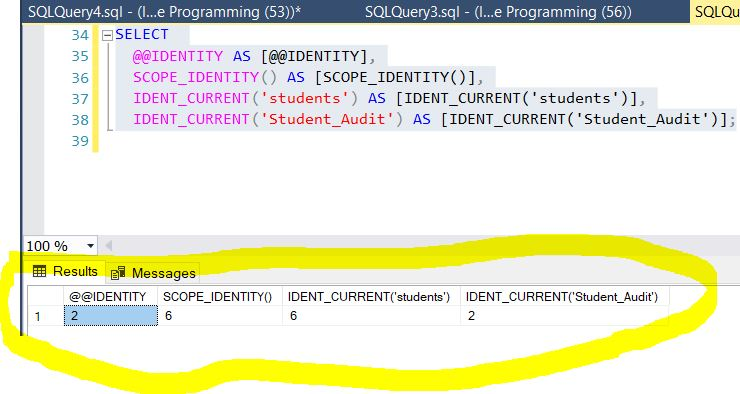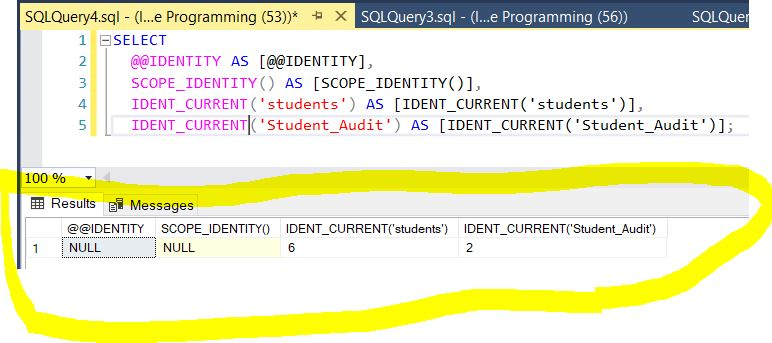﻿ SQL Identity - TutorialsTrend
This Angular tutorial helps you get started with Angular quickly and effectively through many practical examples.
`

# SQL-Identity

In this article, we will see how to use @@IDENTITY, SCOPE_IDENTITY and IDEN_CURRENT in SQL Server. Here, we will see the difference between them. So let's have a look at a practical example.

For that we have three options.

1. @@identity - This function returns the last identity created in the current session and across all scopes.
2. scope_identity - This function returns the last identity created in the current session and the current scope.
3. ident_current - This returns the last identity value generated for a specific table in any session and any scope.

So mainly difference in all three is related to the session and scope. So firstly we will see what are the session and scope in SQL Query.

1. Session - The session is the database connection or Session is the period of time between a client logging in (connecting to) a SQL Server database and the client logging out (exiting) the SQL Server database.
2. Scope - The scope is the current query or the current stored procedure.

#### 1. @@identity

This function returns the last identity created in the current session and across all scopes.

Example

A situation where we create an insert trigger on table which inserts a row in another table with generate an identity column, then @@IDENTITY returns that identity record which is created by trigger.

#### 2. Scope_Identity

This function returns the last identity created in the current session and the current scope.

Example

A situation where we create an insert trigger on table which inserts a row in another table with generate an identity column, then SCOPE_IDENTITY result is not affected

#### 3. Dent_Current

This returns the last identity value generated for a specific table in any session and any scope. In other words - we can say it is not affected by scope and session, it only depends on a particular table and returns that table related identity value which is generated in any session or scope.

I am explaining the above process with the help of some sample SQL Query and Trigger, hope it helps:

Now we create one table called students which have the identity column and insert some data list as below insert query. After that we create a another table named Student_Audit. We create a insert trigger on the student table. That means when we insert any value in the table students. it will automatically insert row in Student_Audit table.

``````Create table students // Creating Students table
(
StudentID int identity(1,1),
StudentName varchar(100),
StudentAge int
)
Go
Insert into students(StudentName, StudentAge) values('Rohatash', 30) // Inserting some data in Students table
Insert into students(StudentName, StudentAge) values('Ritesh', 28)
Insert into students(StudentName, StudentAge) values('Rahul', 27)
Insert into students(StudentName, StudentAge) values('Manoj', 25)
Insert into students(StudentName, StudentAge) values('Raju', 32)
Go
Select * from students
Go
Create table Student_Audit // Creating Student_Audit table
(
Auditid int identity(1,1),
StudentID int,
StudentName varchar(100),
StudentAge int
)
GO
CREATE TRIGGER triggerinset ON students FOR INSERT // Creating triger to Inserting some data in Student_Audit table
AS
BEGIN
INSERT into Student_Audit default values
END;
Go
Go
Insert into students(StudentName, StudentAge) values('Raj', 35) // Now when you insert in the students table trigger will file on table Student_Audit.
GO
SELECT
@@IDENTITY AS [@@IDENTITY],
SCOPE_IDENTITY() AS [SCOPE_IDENTITY()],
IDENT_CURRENT('students') AS [IDENT_CURRENT('students')],
IDENT_CURRENT('Student_Audit') AS [IDENT_CURRENT('Student_Audit')];``````

The following output will show the difference between all three identity.

OutputNow open new query window and paste the following select Query.

``````SELECT
@@IDENTITY AS [@@IDENTITY],
SCOPE_IDENTITY() AS [SCOPE_IDENTITY()],
IDENT_CURRENT('students') AS [IDENT_CURRENT('students')],
IDENT_CURRENT('Student_Audit') AS [IDENT_CURRENT('Student_Audit')];``````

OutputNext